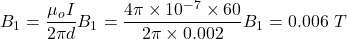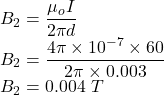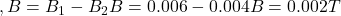Two long, parallel wires separated by 5.0 mm each carry a current of 60 A. These two currents are oppositely directed. What is the magnitude

Question

Two long, parallel wires separated by 5.0 mm each carry a current of 60 A. These two currents are oppositely directed. What is the magnitude of the magnetic field at a point that is between the two wires and 2.0 mm from one of the two wires

in progress 0
5 months 2021-08-28T08:59:40+00:00 2 Answers 0 views 0

0.002T

Explanation:

It is given that,

Distance between two parallel wires, d = 5 mm = 0.005

Current in each wire, I = 60 A

Let B is the magnitude of the magnetic field at a point that is between the two wires and 2.0 mm from one of the two wires.

calculating the magnetic field due to first wire is given by :The magnetic field due to second wire is given by :Let B is the net magnetic field from wire A, so,2. Given Information:

Distance between wires = d = 5 mm = 0.005 m

Current in wires = I = 60 A

Required Information:

Magnetic field at distance 2 mm between two wires  = B = ?

B = 0.010 T

Explanation:

When two parallel wires carrying a current I are separated by a distance d then the magnetic field is given by

B1 = μ₀I/2πd1

B2 = μ₀I/2πd2

Where μ₀= 4πx10⁻⁷ is the permeability of free space

The overall magnitude of magnetic field is

B = B1 + B2

Since the two wires are separated by 0.005 m then

d1 = 0.005 – 0.002 = 0.003 m

d2 = 0.002 m

B1 = 4πx10⁻⁷*60/2π*0.003

B1 = 0.004 T

B2 = 4πx10⁻⁷*60/2π*0.002

B2 = 0.006 T

B = 0.004 + 0.006

B = 0.010 T

Therefore, the magnitude of the magnetic field at a point between two wires and 0.002 m away from one of wire is 0.010 T